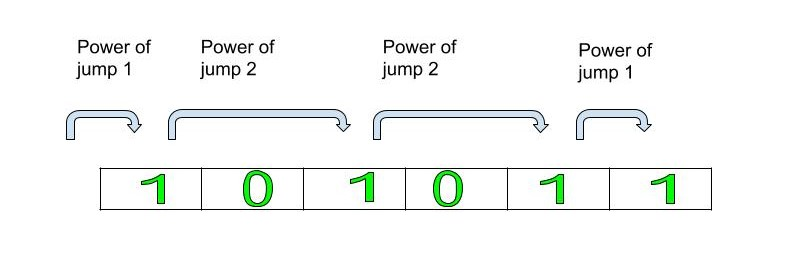# Maximum power of jump required to reach the end of string

• Difficulty Level : Medium
• Last Updated : 27 Jul, 2021

Given a string consisting of 1 and 0, the task is to find out the maximum power of jump required to reach the end of the string. At a time you can jump from one 1 to next 1 or from one 0 to next 0
Note: Power of jump is defined as the distance between two consecutive 1’s or two consecutive 0’s.
Examples:

```Input: 10101
Output: 2
First, make a power jump of 1 to reach first 1,
then a power jump of 2 to reach second 1
and then finally a power jump of 2
to reach the end of the string
hence the maximum power of jump is 2.

Input: 11110
Output: 5
Since to reach the end of the string, we have to make
power jump of 5 to reach 0 and end of the string```Approach:

• Check if the last character of given string is 1 or 0.
• If the last character is 1 then search for the first 1 in the string and continue to jump to the next 1 to reach the last of the string. Update the maximum jumps.
• Similarly, if the last character is 0 then search for the first 0 in the string and continue to jump to the next 0 to reach the last of the string. Update the maximum jumps.
• Return the maximum number of jumps taken to reach the end.

## C++

 `// C++ program to calculate power of jump``#include``using` `namespace` `std;` `// Function to calculate the maximum power of the jump``int` `powerOfJump(string s)``{``    ``// Initialize the count with 1``    ``int` `count = 1;``    ``int` `max_so_far = INT_MIN;``    ` `    ``// Find the character at last index``    ``char` `ch = s[s.length() - 1];``    ` `    ``// Start traversing the string``    ``for` `(``int` `i = 0; i < s.length(); i++)``    ``{``        ``// Check if the current char is equal``        ``// to the last character``        ``if` `(s[i] == ch)``        ``{``            ``// max_so_far stores maximum value of``            ``// the power of the jump from starting``            ``// to ith position``            ``if` `(count > max_so_far) {``                ``max_so_far = count;``                ` `            ``}``            ``// Reset the count to 1``            ``count = 1;``            ` `        ``}``        ``// Else, increment the number of jumps/count``        ``else``        ``count++;``        ` `    ``}``    ``// Return the maximum number of jumps``    ``return` `max_so_far;``    ` `}` `// Driver code``int` `main(){``    ``string st = ``"1010101"``;``    ``cout<

## Java

 `// java program to calculate power of jump``import` `java.util.ArrayList;` `public` `class` `string_sort {` `    ``// Function to calculate the maximum power of the jump``    ``public` `static` `int` `powerOfJump(String s)``    ``{``        ``// Initialize the count with 1``        ``int` `count = ``1``;``        ``int` `max_so_far = Integer.MIN_VALUE;` `        ``// Find the character at last index``        ``char` `ch = s.charAt(s.length() - ``1``);` `        ``// Start traversing the string``        ``for` `(``int` `i = ``0``; i < s.length(); i++) {` `           ``// Check if the current char is equal``           ``// to the last character``            ``if` `(s.charAt(i) == ch) {` `                ``// max_so_far stores maximum value of``                ``// the power of the jump from starting``                ``// to ith position``                ``if` `(count > max_so_far) {``                    ``max_so_far = count;``                ``}``                ` `                ``// Reset the count to 1``                ``count = ``1``;``            ``}` `            ``// Else, increment the number of jumps/count``            ``else``                ``count++;``        ``}` `        ``// Return the maximum number of jumps``        ``return` `max_so_far;``    ``}``    ``// Driver code``    ``public` `static` `void` `main(String[] args)``    ``{``        ``String st = ``"1010101"``;``        ``System.out.println(powerOfJump(st));``    ``}``}`

## Python3

 `# Python3 program to calculate``# power of jump` `# Function to calculate the maximum``# power of the jump``def` `powerOfJump(s):` `    ``# Initialize the count with 1``    ``count ``=` `1``    ``max_so_far ``=` `0``    ` `    ``# Find the character at last index``    ``ch ``=` `s[``-``1``]``    ` `    ``# Start traversing the string``    ``for` `i ``in` `range``(``0``, ``len``(s)):``    ` `        ``# Check if the current char is``        ``# equal to the last character``        ``if` `s[i] ``=``=` `ch:``        ` `            ``# max_so_far stores maximum value of``            ``# the power of the jump from starting``            ``# to ith position``            ``if` `count > max_so_far:``                ``max_so_far ``=` `count``            ` `            ``# Reset the count to 1``            ``count ``=` `1``        ` `        ``# Else, increment the number``        ``# of jumps/count``        ``else``:``            ``count ``+``=` `1``    ` `    ``# Return the maximum number of jumps``    ``return` `max_so_far` `# Driver Code``if` `__name__ ``=``=` `"__main__"``:` `    ``st ``=` `"1010101"``    ``print``(powerOfJump(st))``    ` `# This code is contributed``# by Rituraj Jain`

## C#

 `// C# program to calculate``// power of jump``using` `System;` `class` `GFG``{` `// Function to calculate the``// maximum power of the jump``public` `static` `int` `powerOfJump(String s)``{``    ``// Initialize the count with 1``    ``int` `count = 1;``    ``int` `max_so_far = ``int``.MinValue;` `    ``// Find the character at last index``    ``char` `ch = s[s.Length - 1];` `    ``// Start traversing the string``    ``for` `(``int` `i = 0; i < s.Length; i++)``    ``{` `    ``// Check if the current char is``    ``// equal to the last character``        ``if` `(s[i] == ch)``        ``{` `            ``// max_so_far stores maximum value``            ``// of the power of the jump from``            ``// starting to ith position``            ``if` `(count > max_so_far)``            ``{``                ``max_so_far = count;``            ``}``            ` `            ``// Reset the count to 1``            ``count = 1;``        ``}` `        ``// Else, increment the number``        ``// of jumps/count``        ``else``            ``count++;``    ``}` `    ``// Return the maximum number of jumps``    ``return` `max_so_far;``}` `// Driver code``public` `static` `void` `Main()``{``    ``String st = ``"1010101"``;``    ``Console.WriteLine(powerOfJump(st));``}``}` `// This code is contributed``// by Akanksha Rai(Abby_akku)`

## PHP

 ` ``\$max_so_far``)``            ``{``                ``\$max_so_far` `= ``\$count``;   ``            ``}``            ` `            ``// Reset the count to 1``            ``\$count` `= 1;``            ` `        ``}``        ` `        ``// Else, increment the number``        ``// of jumps/count``        ``else``        ``\$count``++;``    ``}``    ` `    ``// Return the maximum number``    ``// of jumps``    ``return` `\$max_so_far``;``}` `// Driver code``\$st` `= ``"1010101"``;``echo` `powerOfJump(``\$st``);``    ` `// This code is contributed by ajit``?>`

## Javascript

 ``
Output:
`2`

Time Complexity : O(n) where n is the length of the string

My Personal Notes arrow_drop_up